# Welcome To Big Board Facts NEXT NEXT NEXT

• Slides: 61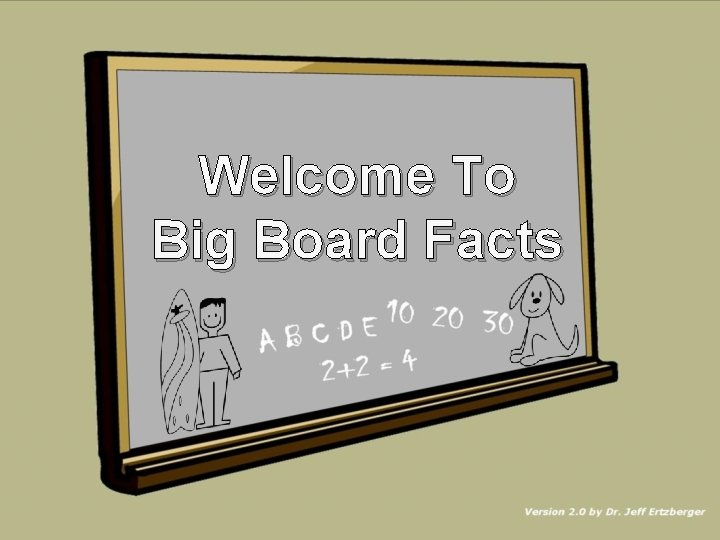Welcome To Big Board FactsNEXTNEXT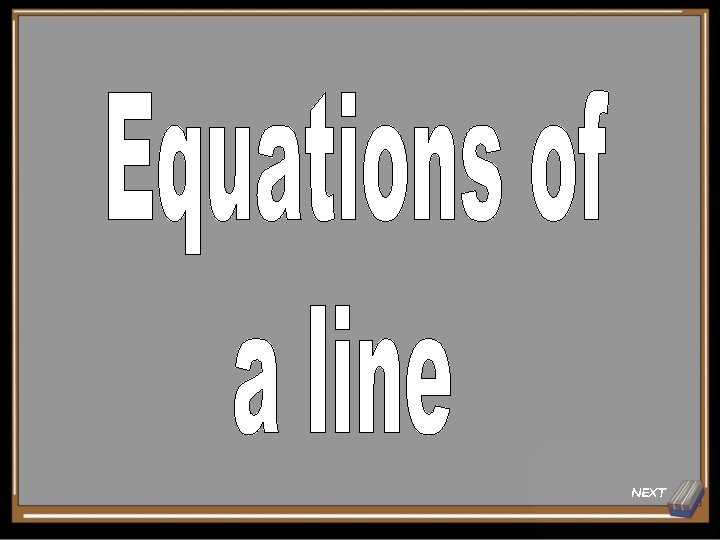NEXT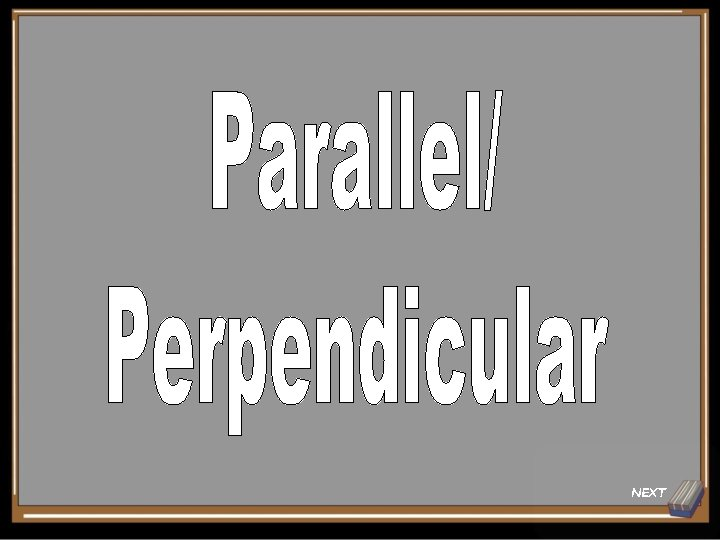NEXTNEXTNEXT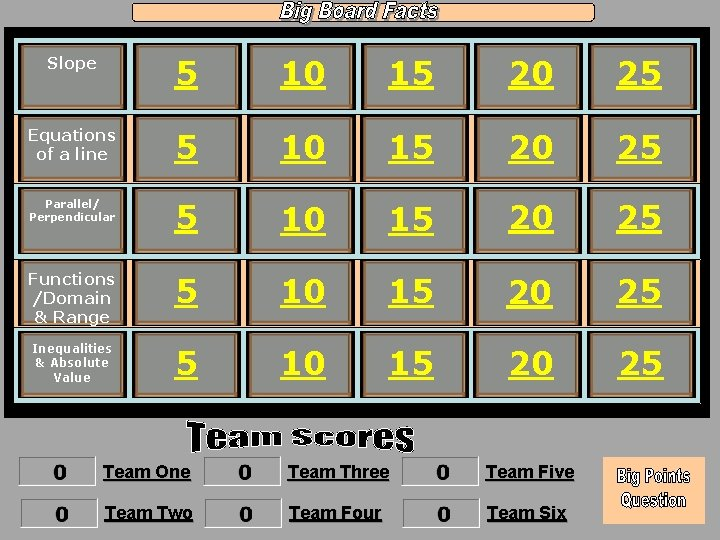Slope 5 10 15 20 25 Equations of a line 5 10 15 20 25 Parallel/ Perpendicular 5 10 15 20 25 Functions /Domain & Range 5 10 15 20 25 Inequalities & Absolute Value 5 10 15 20 25 Team One Team Three Team Five Team Two Team Four Team Six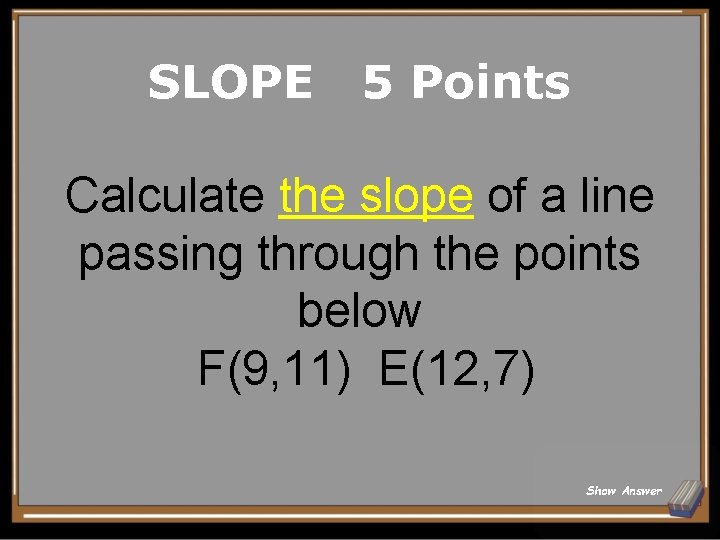SLOPE 5 Points Calculate the slope of a line passing through the points below F(9, 11) E(12, 7) Show Answer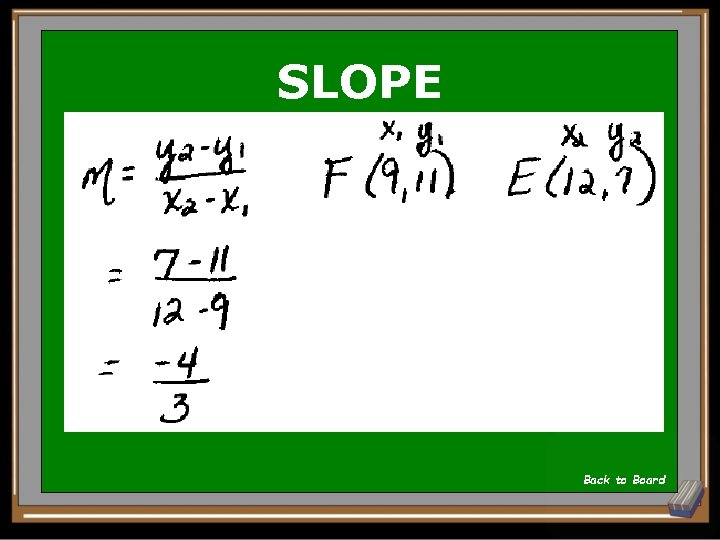SLOPE Back to BoardSLOPE 10 Points Calculate the slope from the graph below Show Answer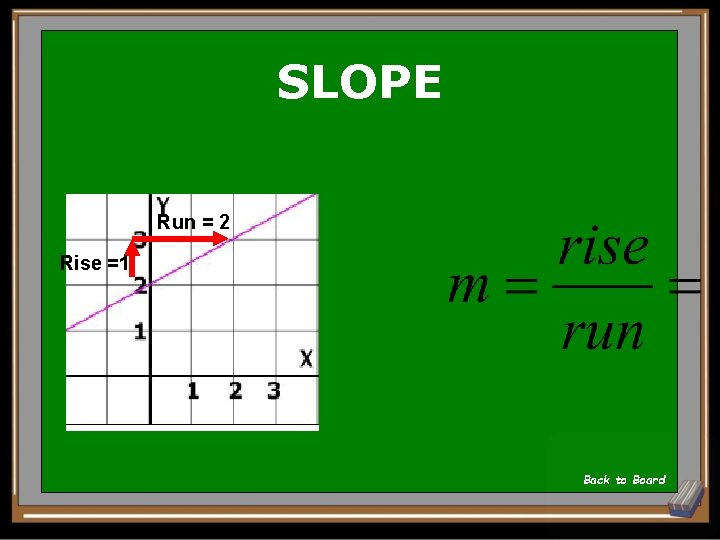SLOPE Run = 2 Rise =1 Back to Board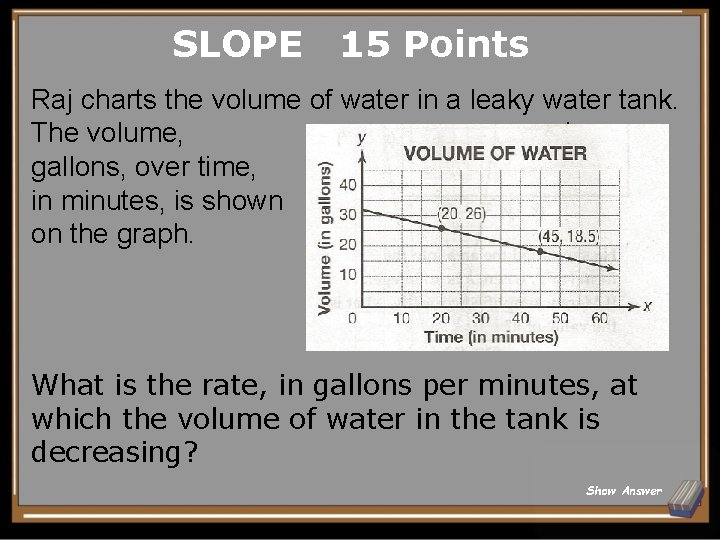SLOPE 15 Points Raj charts the volume of water in a leaky water tank. The volume, in gallons, over time, in minutes, is shown on the graph. What is the rate, in gallons per minutes, at which the volume of water in the tank is decreasing? Show AnswerSLOPE -0. 3 gallons per minute Back to Board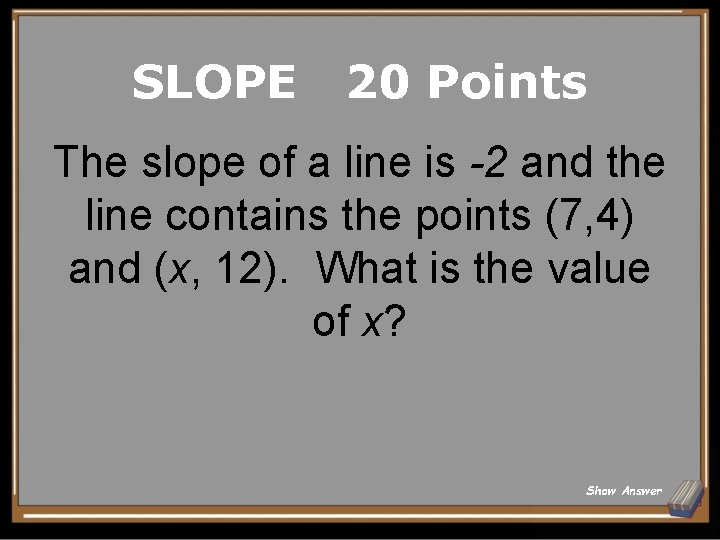SLOPE 20 Points The slope of a line is -2 and the line contains the points (7, 4) and (x, 12). What is the value of x? Show Answer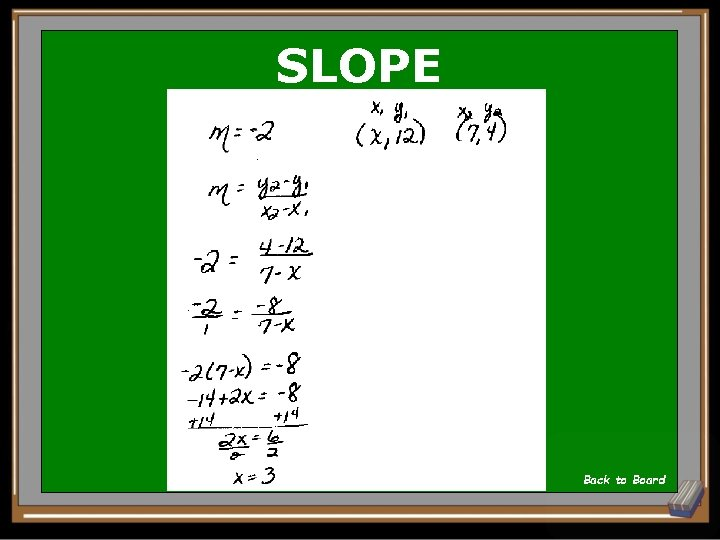SLOPE 3 Back to Board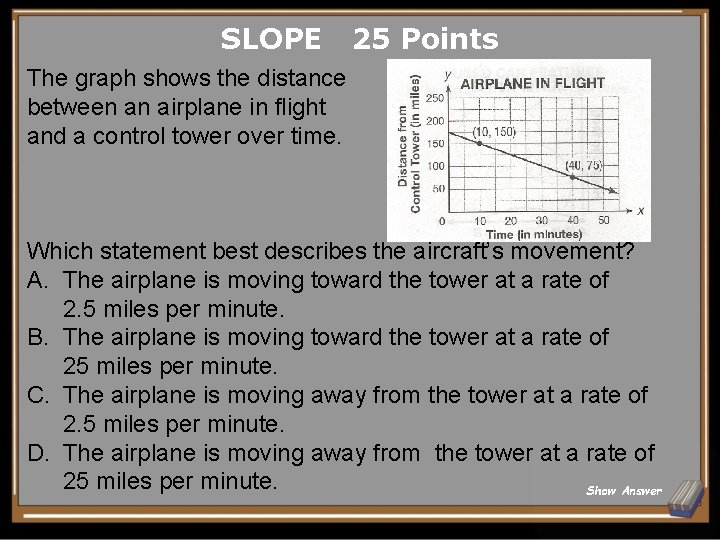SLOPE 25 Points The graph shows the distance between an airplane in flight and a control tower over time. Which statement best describes the aircraft’s movement? A. The airplane is moving toward the tower at a rate of 2. 5 miles per minute. B. The airplane is moving toward the tower at a rate of 25 miles per minute. C. The airplane is moving away from the tower at a rate of 2. 5 miles per minute. D. The airplane is moving away from the tower at a rate of 25 miles per minute. Show AnswerSLOPE A Back to Board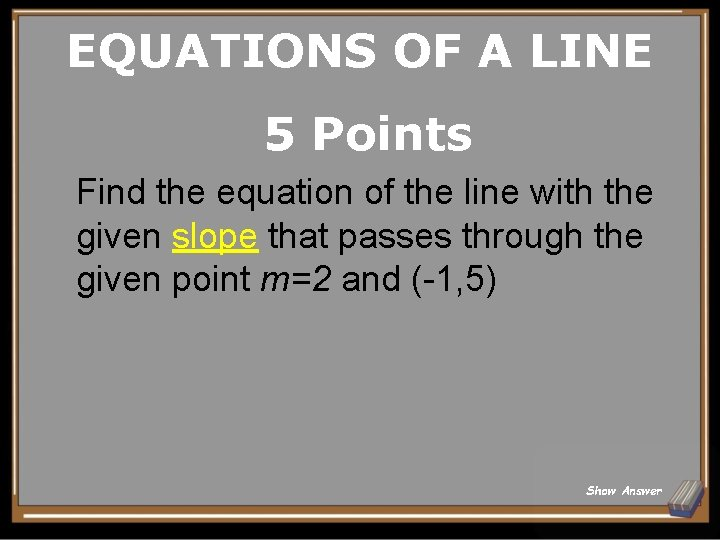EQUATIONS OF A LINE 5 Points Find the equation of the line with the given slope that passes through the given point m=2 and (-1, 5) Show Answer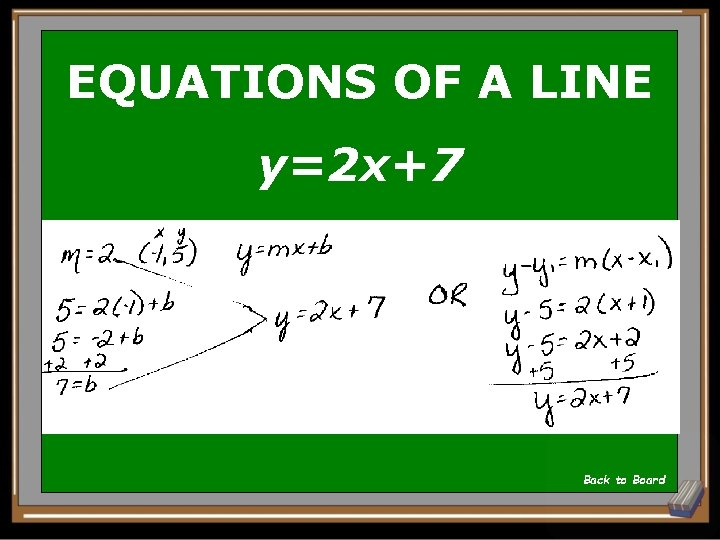EQUATIONS OF A LINE y=2 x+7 Back to BoardEQUATIONS OF A LINE 10 Points Write the equation of the line graphed below. Express your answer in standard form. Show Answer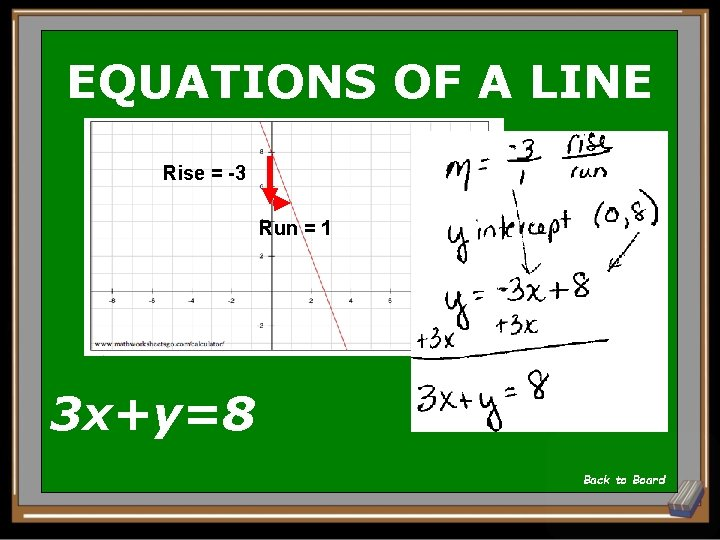EQUATIONS OF A LINE Rise = -3 Run = 1 3 x+y=8 Back to BoardEQUATIONS OF A LINE 15 Points Find the equation of the line that passes through the following two points: (1, 12) and (-3, 5) Express your answer in slope intercept form. Show AnswerEQUATIONS OF A LINE Back to Board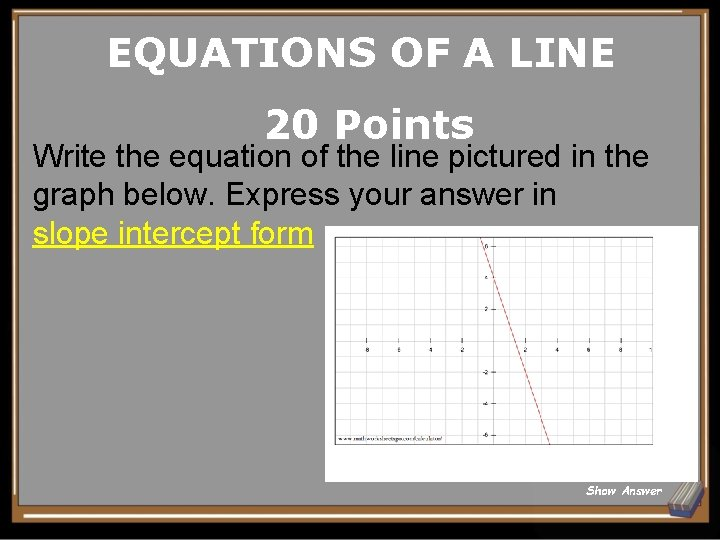EQUATIONS OF A LINE 20 Points Write the equation of the line pictured in the graph below. Express your answer in slope intercept form Show Answer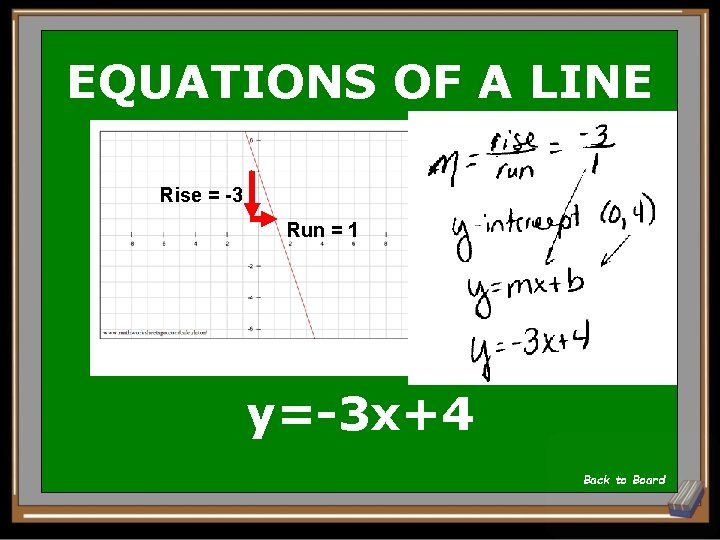EQUATIONS OF A LINE Rise = -3 Run = 1 y=-3 x+4 Back to BoardEQUATIONS OF A LINE 25 Points Convert the point slope equation to slope intercept form. y-5=6(x-8) Show Answer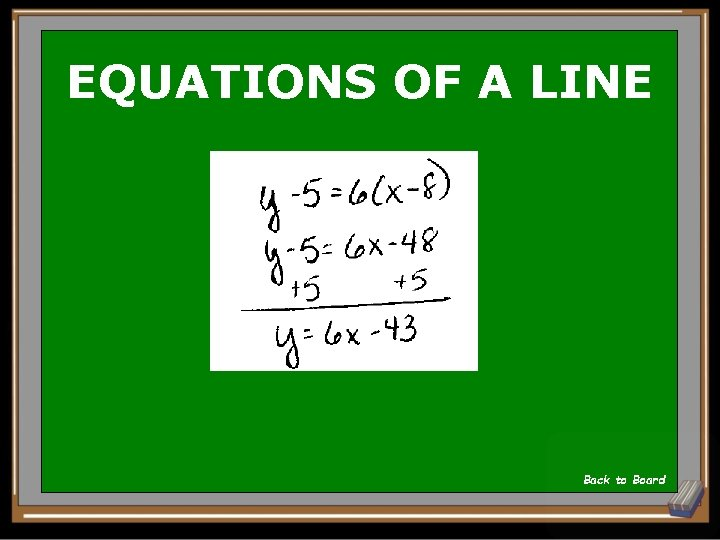EQUATIONS OF A LINE y=6 x-43 Back to Board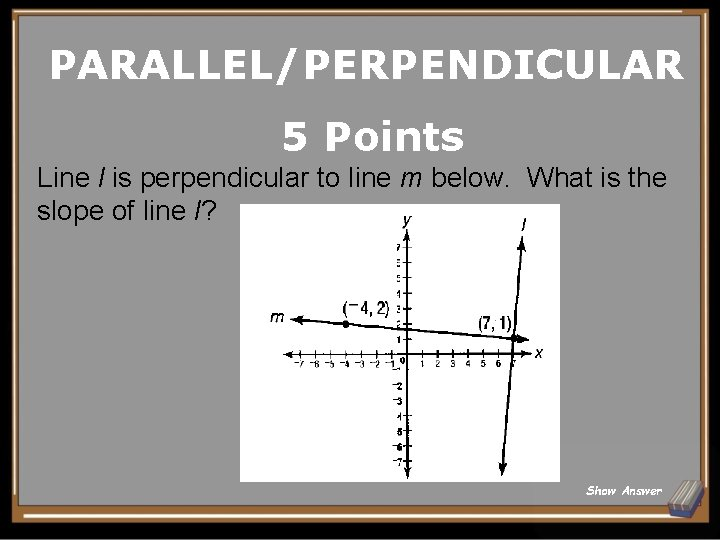PARALLEL/PERPENDICULAR 5 Points Line l is perpendicular to line m below. What is the slope of line l? Show AnswerPARALLEL/PERPENDICULAR Back to Board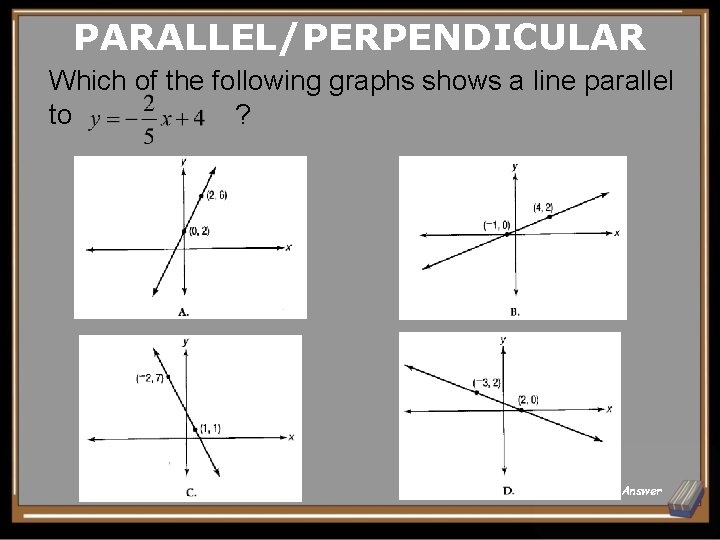PARALLEL/PERPENDICULAR Which of the following graphs shows a line parallel to ? Show AnswerPARALLEL/PERPENDICULAR D Back to BoardPARALLEL/PERPENDICULAR On the coordinate grid below, line l is parallel to What is the slope of line l? Show Answer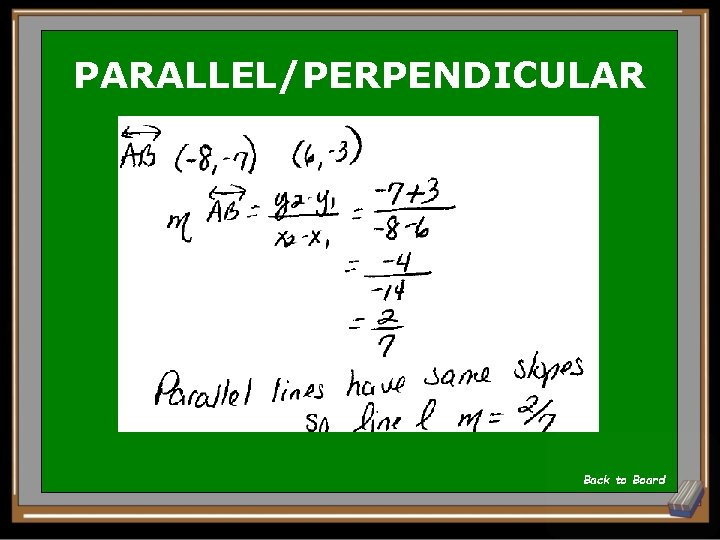PARALLEL/PERPENDICULAR Back to BoardPARALLEL/PERPENDICULAR Yael graphs line on a coordinate grid through points A(-10, 4) and B(5, 3). If is perpendicular to , what is the slope of ? Show AnswerPARALLEL/PERPENDICULAR 15 Back to Board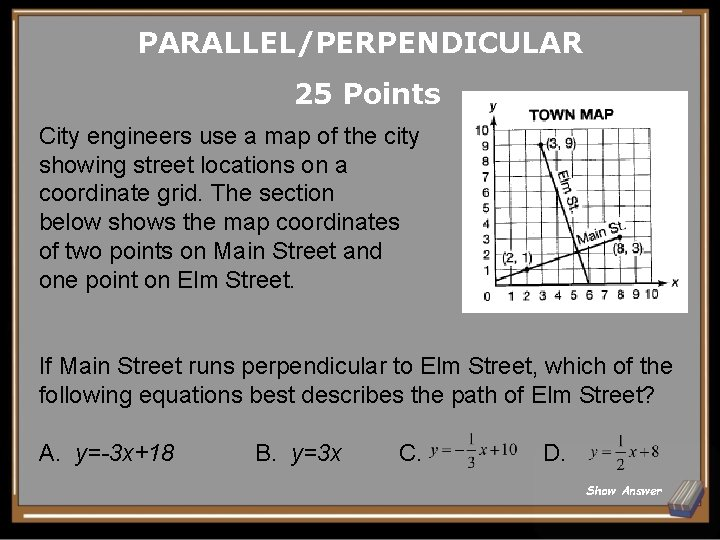PARALLEL/PERPENDICULAR 25 Points City engineers use a map of the city showing street locations on a coordinate grid. The section below shows the map coordinates of two points on Main Street and one point on Elm Street. If Main Street runs perpendicular to Elm Street, which of the following equations best describes the path of Elm Street? A. y=-3 x+18 B. y=3 x C. D. Show AnswerPARALLEL/PERPENDICULAR A Back to BoardFUNCTIONS/DOMAIN & RANGE 5 Points Which set of ordered pairs does NOT represent a function? A. B. C. D. {(4, 8), (1, 8), (5, 11), (0, 0)} {(2, 1), (1, 2), (2, 0), (5, 5)} {(0, 0), (1, 1), (2, 4), (3, 9)} {(1, 7), (4, 7), (7, 7), (10, 7)} Show AnswerFUNCTIONS/DOMAIN & RANGE B Back to Board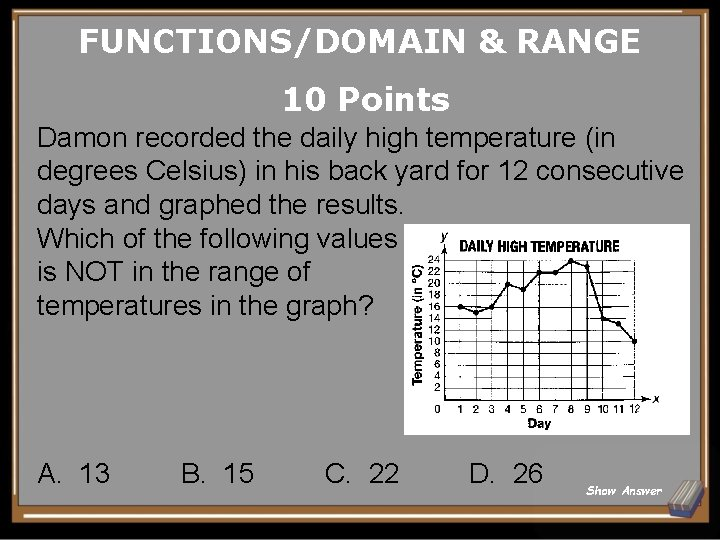FUNCTIONS/DOMAIN & RANGE 10 Points Damon recorded the daily high temperature (in degrees Celsius) in his back yard for 12 consecutive days and graphed the results. Which of the following values is NOT in the range of temperatures in the graph? A. 13 B. 15 C. 22 D. 26 Show Answer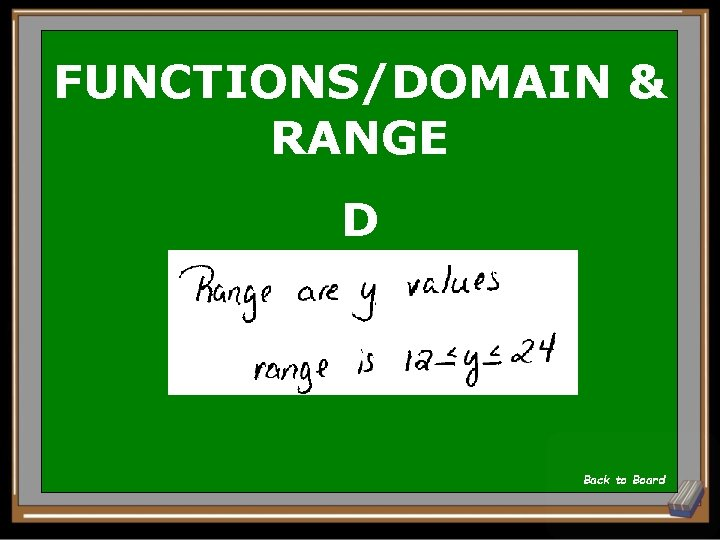FUNCTIONS/DOMAIN & RANGE D Back to Board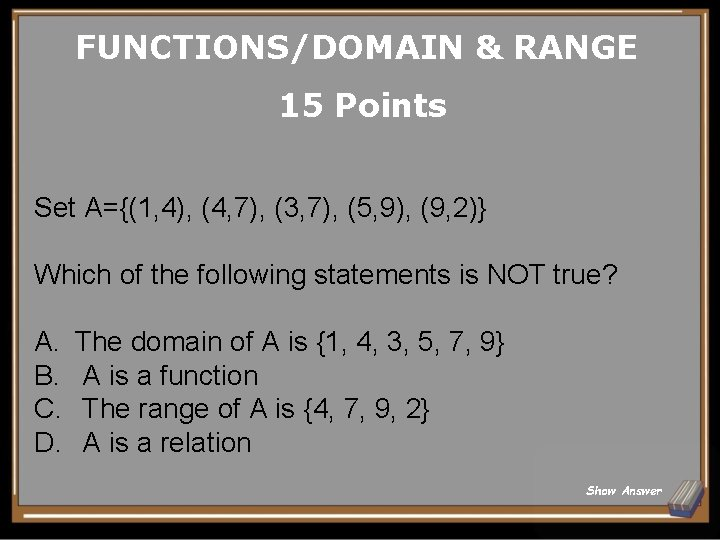FUNCTIONS/DOMAIN & RANGE 15 Points Set A={(1, 4), (4, 7), (3, 7), (5, 9), (9, 2)} Which of the following statements is NOT true? A. B. C. D. The domain of A is {1, 4, 3, 5, 7, 9} A is a function The range of A is {4, 7, 9, 2} A is a relation Show Answer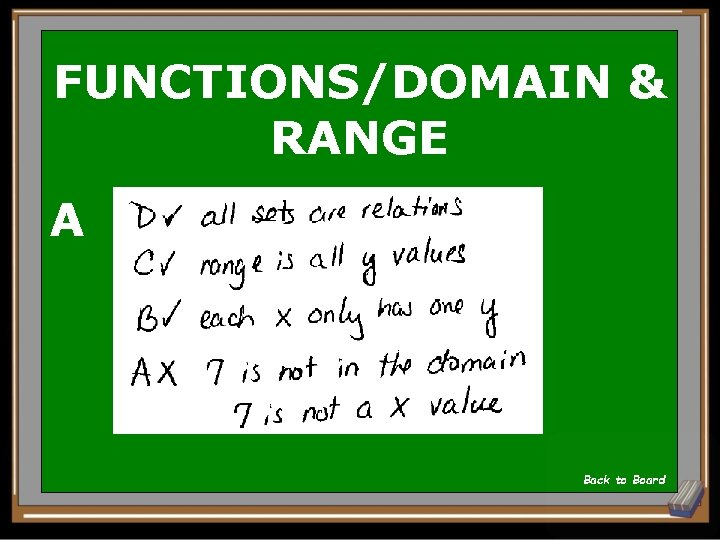FUNCTIONS/DOMAIN & RANGE A Back to BoardFUNCTIONS/DOMAIN & RANGE 20 Points If {-3, -1, 1, 2, 3} is the domain, what is the range of A. B. C. D. {-3, -1, 1, 2} {0, 2, 6, 12} {1, 4, 9} {-3, -2, -1, 1} Show Answer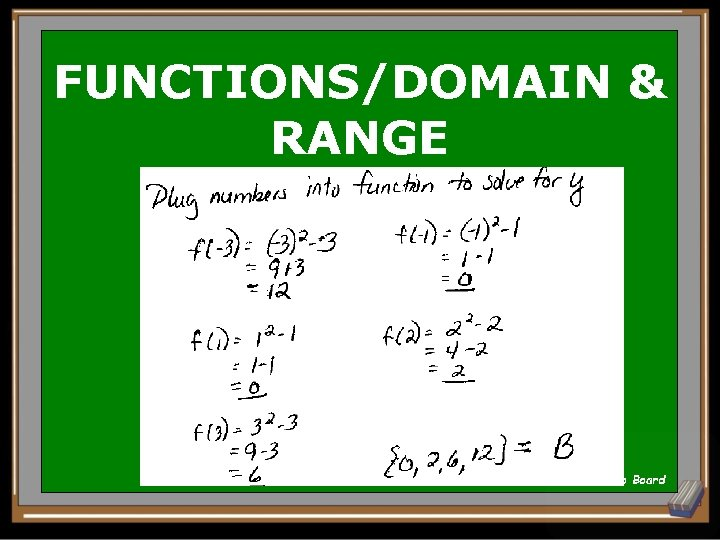FUNCTIONS/DOMAIN & RANGE B Back to BoardFUNCTIONS/DOMAIN & RANGE 25 Points A farmer uses a large cylindrical water tank for irrigation. When the farmer waters his fields, the depth in the tank decreases at a steady rate of 0. 3 inch per minute. After 20 minutes of watering, the depth of the water in the tank is 9 inches. Which graph shows the relationship between d, the depth of the water in inches, and t, the time in minutes? A. B. C. Show AnswerFUNCTIONS/DOMAIN & RANGE B At x=20 y value is at 9 Back to Board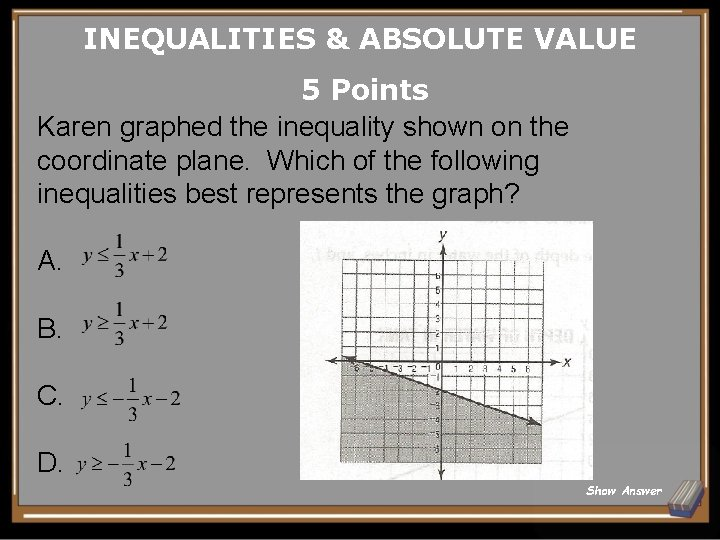INEQUALITIES & ABSOLUTE VALUE 5 Points Karen graphed the inequality shown on the coordinate plane. Which of the following inequalities best represents the graph? A. B. C. D. Show Answer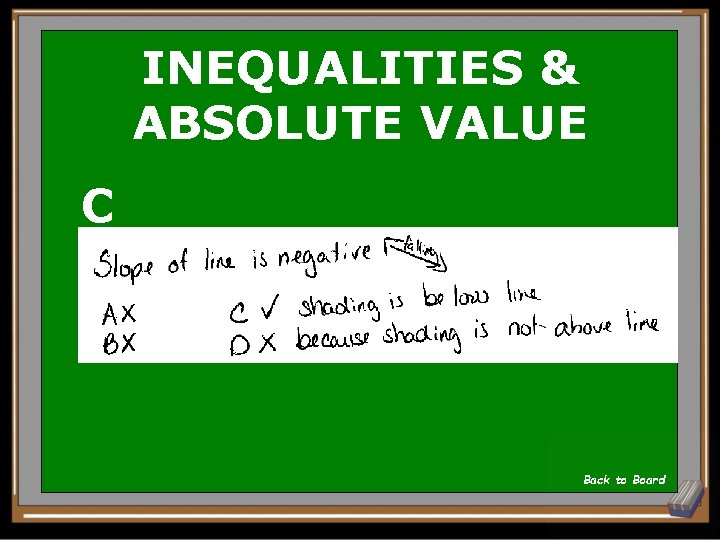INEQUALITIES & ABSOLUTE VALUE C Back to Board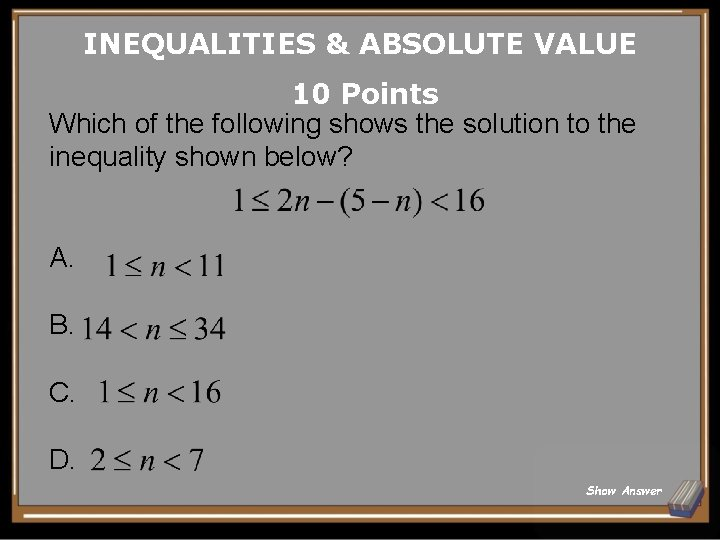INEQUALITIES & ABSOLUTE VALUE 10 Points Which of the following shows the solution to the inequality shown below? A. B. C. D. Show Answer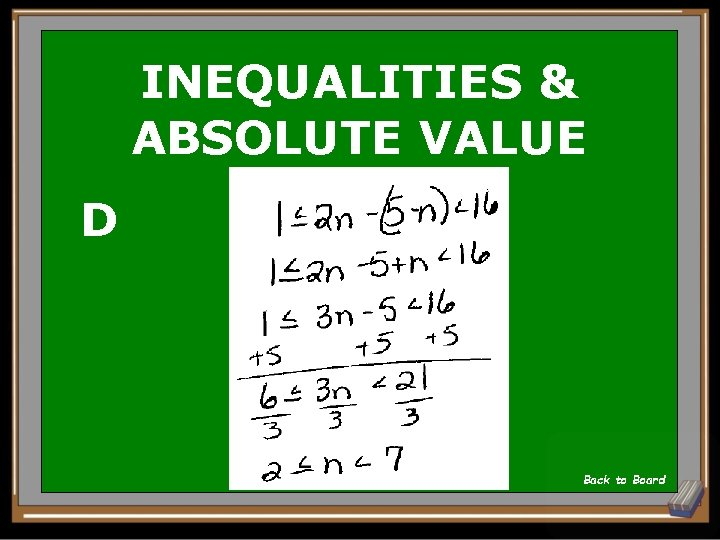INEQUALITIES & ABSOLUTE VALUE D Back to BoardINEQUALITIES & ABSOLUTE VALUE 15 Points The graph shows a system of linear inequalities. Which of the following (x, y) coordinate pairs is NOT a solution to the system of inequalities? A. (0, 0) B. (0, 6) C. (-5, 0) D. (0, -2) Show AnswerINEQUALITIES & ABSOLUTE VALUE Solutions are in the shaded region or on a SOLID line X A in shaded region √ B on dotted line X C in shaded region X D on solid line Back to Board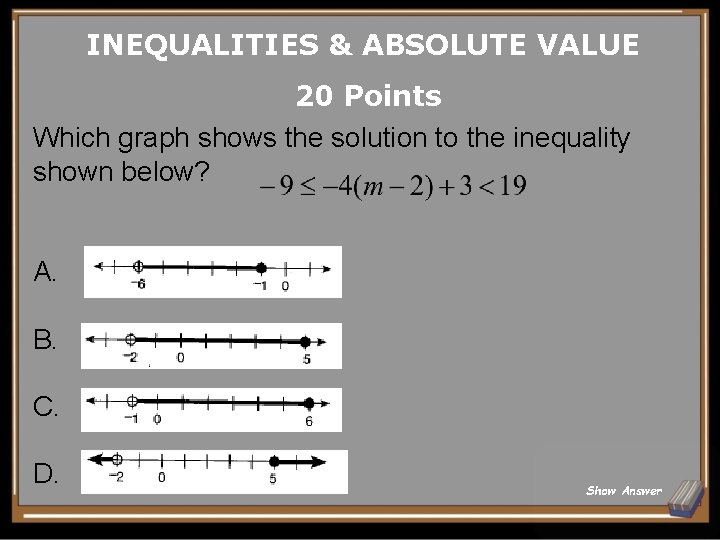INEQUALITIES & ABSOLUTE VALUE 20 Points Which graph shows the solution to the inequality shown below? A. B. C. D. Show Answer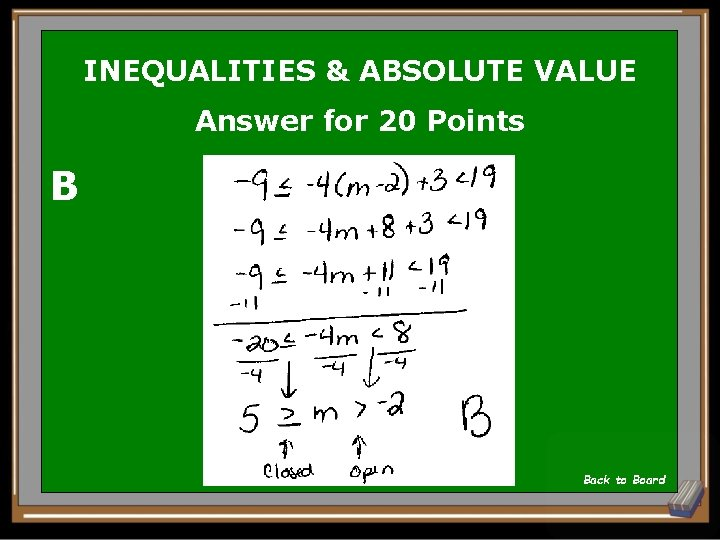INEQUALITIES & ABSOLUTE VALUE Answer for 20 Points B Back to BoardINEQUALITIES & ABSOLUTE VALUE Solve the absolute value inequality Show Answer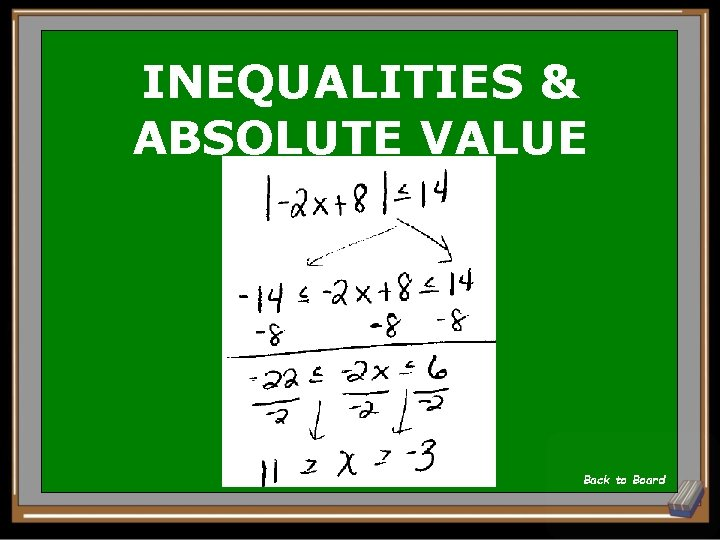INEQUALITIES & ABSOLUTE VALUE Back to Board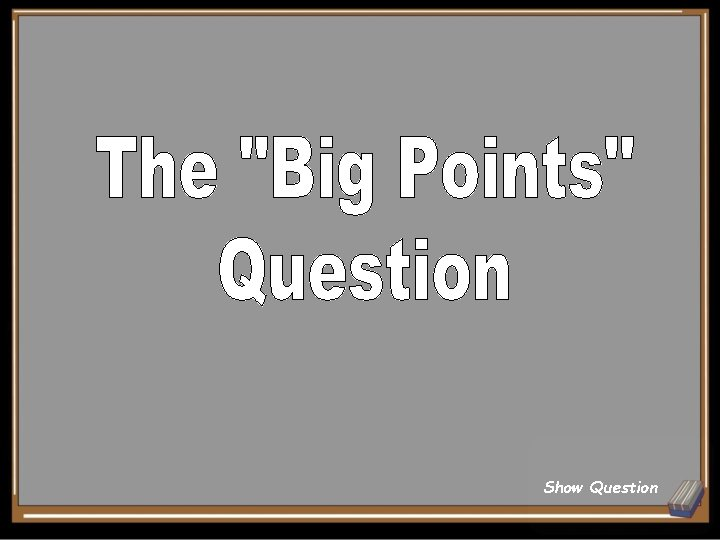Show Question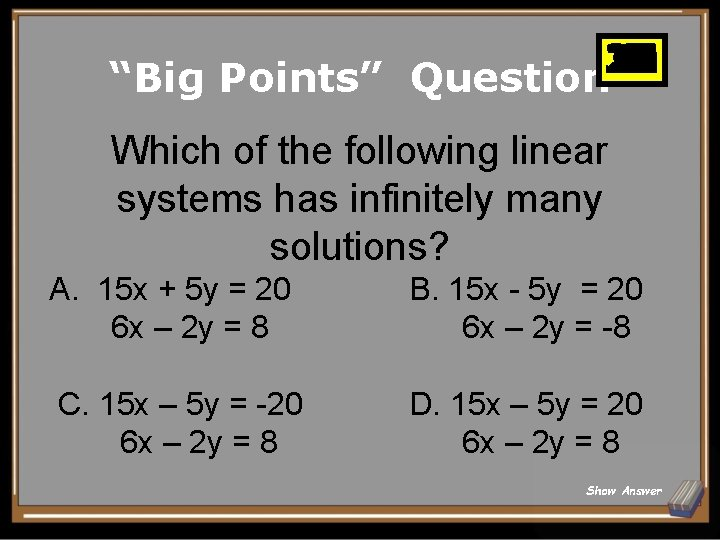25 26 27 28 29 30 10 11 12 13 14 15 16 17 18 19 20 21 22 23 24 1 2 3 4 5 6 7 8 9 “Big Points” Question Which of the following linear systems has infinitely many solutions? A. 15 x + 5 y = 20 6 x – 2 y = 8 B. 15 x - 5 y = 20 6 x – 2 y = -8 C. 15 x – 5 y = -20 6 x – 2 y = 8 D. 15 x – 5 y = 20 6 x – 2 y = 8 Show AnswerBig Points Answer for Big Points D Back to Board